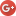# Kuwait Distance Calculator - Distance from one place to another in Kuwait.Calculate Kuwait Distances Below

Tip: Entering something like London to Moscow gets results whereas " London Moscow " will not.

### Popular Distances and Towns for Kuwait on Distance Calculator

Distances Calculated For Kuwait By Our Visitors

Please note, results are based on user searches for Kuwait and classifications provided by our data suppliers. We do our best to ensure accuracy, do please tell us if you notice anything odd!

The is 18 miles in a straight line. (Ahmadi is in Kuwait, Salmiya is in Kuwait )

The is 7 miles in a straight line. (Kuwait is in Kuwait, Rumaithiya is in Kuwait )

The is 7 miles in a straight line. (Salmiyah is in Kuwait, Khaitan is in Al Asimah, Kuwait )

The is 12 miles in a straight line. (Fintas is in Kuwait, Hawalli is in Al Asimah, Kuwait )

The is 2 miles in a straight line. (Abrak Khetan is in Kuwait, South Surra is in Al Asimah, Kuwait )

The is 4 miles in a straight line. (Shuwaikh is in Kuwait, Rowda is in Al Asimah, Kuwait )

The is 11 miles in a straight line. (Abraq Khaitan is in Kuwait, Manifah is in Al Asimah, Kuwait )

The is 5 miles in a straight line. (Koweit is in Kuwait, Abrak Khetan is in Al Asimah, Kuwait )

The is 14 miles in a straight line. (Doha is in Kuwait, Bayan is in Ad Dawhah, Kuwait )

The is 16 miles in a straight line. (Al Farwaniyah is in Kuwait, Al-mangaf is in Al Asimah, Kuwait )

The is 15 miles in a straight line. (Al-mangaf is in Kuwait, Janub As Surrah is in Al Asimah, Kuwait )

The is 15 miles in a straight line. (Shuwaikh Port is in Kuwait, Manifah is in Al Asimah, Kuwait )

The is 4 miles in a straight line. (Hawalli is in Kuwait, Salwa is in Al Asimah, Kuwait )

The is 24 miles in a straight line. (Fahaheel is in Kuwait, Sulaibikhat is in Al Asimah, Kuwait )

The is 17 miles in a straight line. (Jahrah is in Kuwait, Jalib Ash Shuyukh is in Al Asimah, Kuwait )

The is 4 miles in a straight line. (Shuaiba is in Kuwait, Ahmadi is in Al Asimah, Kuwait )

The is 4 miles in a straight line. (Ahmad is in Kuwait, Shuaiba is in Al Asimah, Kuwait )

The is 3 miles in a straight line. (Jabriya is in Kuwait, Dasma is in Al Asimah, Kuwait )

The is 2 miles in a straight line. (Al-farawaniyah is in Kuwait, Jalib Ash Shuyukh is in Al Asimah, Kuwait )

The is 2 miles in a straight line. (Al Kuwait is in Kuwait, Kayfan is in Al Asimah, Kuwait )

We have locations the length and breadth of Kuwait with information about provinces, localaties around the outskirts of major cities and radii distances around towns in some of the most obscure outposts of places on the earth. Do check out a few of our other pages and come back soon!Need to know towns within a specific radius of other places try this radius tool